# Last Lecture The thermodynamics of polymer phase separation

• Slides: 45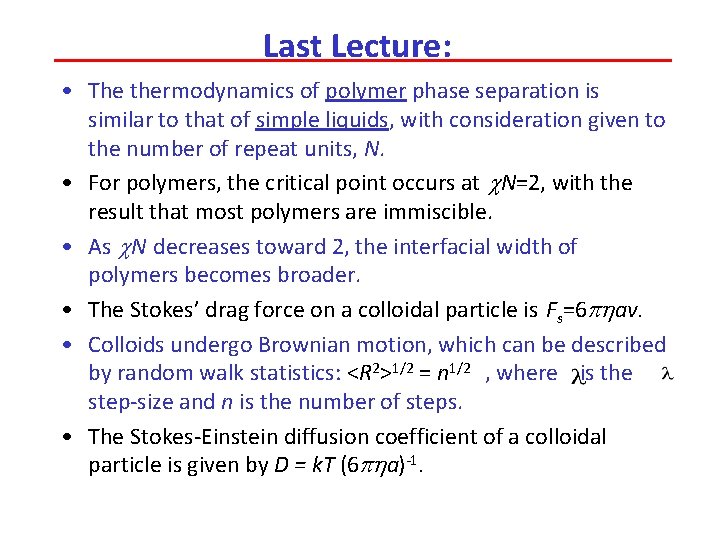Last Lecture: • The thermodynamics of polymer phase separation is similar to that of simple liquids, with consideration given to the number of repeat units, N. • For polymers, the critical point occurs at c. N=2, with the result that most polymers are immiscible. • As c. N decreases toward 2, the interfacial width of polymers becomes broader. • The Stokes’ drag force on a colloidal particle is Fs=6 phav. • Colloids undergo Brownian motion, which can be described by random walk statistics: <R 2>1/2 = n 1/2 , where is the step-size and n is the number of steps. • The Stokes-Einstein diffusion coefficient of a colloidal particle is given by D = k. T (6 pha)-1.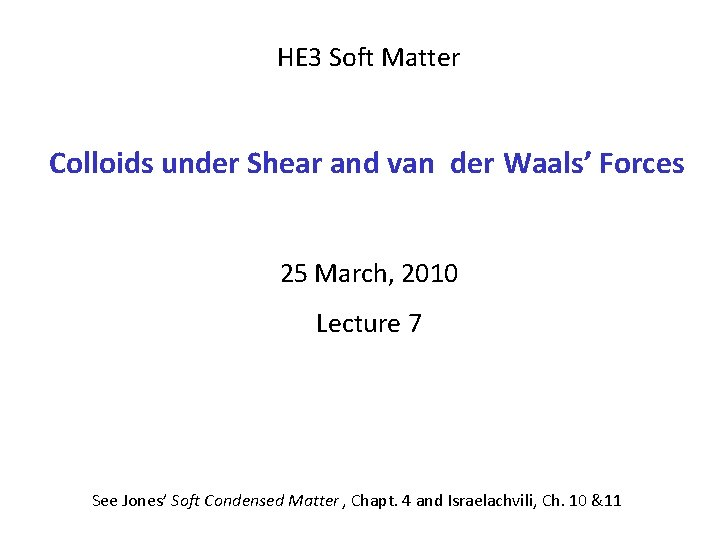HE 3 Soft Matter Colloids under Shear and van der Waals’ Forces 25 March, 2010 Lecture 7 See Jones’ Soft Condensed Matter , Chapt. 4 and Israelachvili, Ch. 10 &11Flow of Dilute Colloidal Dispersions The flow of a dilute colloidal dispersion is Newtonian (i. e. shear strain rate and shear stress are related by a constant h). In a dispersion with a volume fraction of particles of f in a continuous liquid with viscosity ho, the dispersion’s h is given by a series expression proposed by Einstein: A typical value for the constant b is 2. 5; the series can usually be truncated after the first two or three terms, since f must be << 1 for the equation to hold.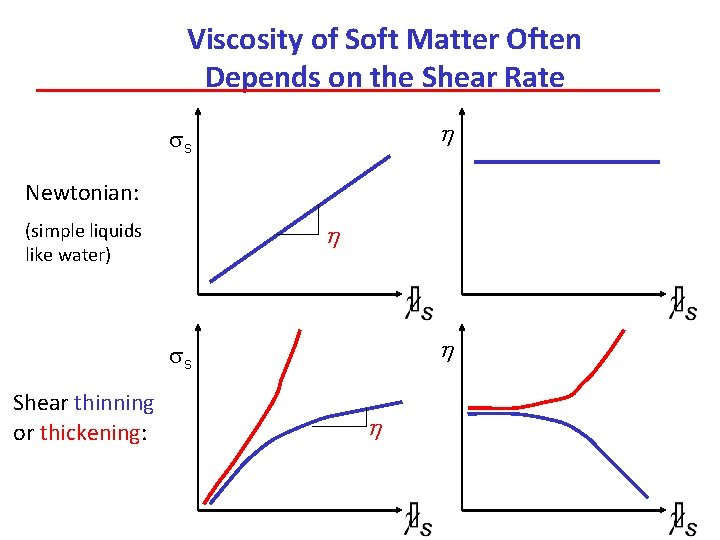Viscosity of Soft Matter Often Depends on the Shear Rate h ss Newtonian: h (simple liquids like water) h ss Shear thinning or thickening: h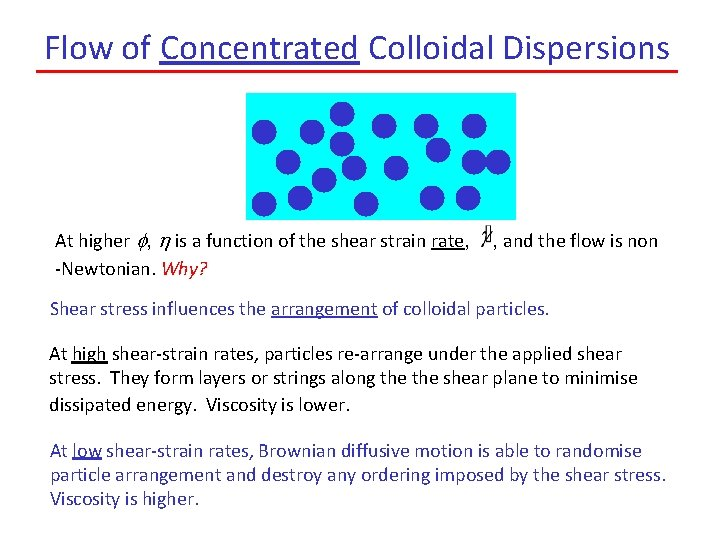Flow of Concentrated Colloidal Dispersions At higher f, h is a function of the shear strain rate, -Newtonian. Why? , and the flow is non Shear stress influences the arrangement of colloidal particles. At high shear-strain rates, particles re-arrange under the applied shear stress. They form layers or strings along the shear plane to minimise dissipated energy. Viscosity is lower. At low shear-strain rates, Brownian diffusive motion is able to randomise particle arrangement and destroy any ordering imposed by the shear stress. Viscosity is higher.Effects of Shear Stress on Colloidal Dispersions With no shear Under a shear stress Confocal Microscope Images MRS Bulletin, Feb 2004, p. 88The Characteristic Time for Shear Ordering, t. S Both the shear strain rate and the Brownian diffusion are associated with a characteristic time, t. A Dx y g v F A The characteristic time for the shear strain, ts, is simply: Slower shear strain rates thus have longer characteristic shear times. One can think of ts as the time over which the particles are redistributed under the shear stress.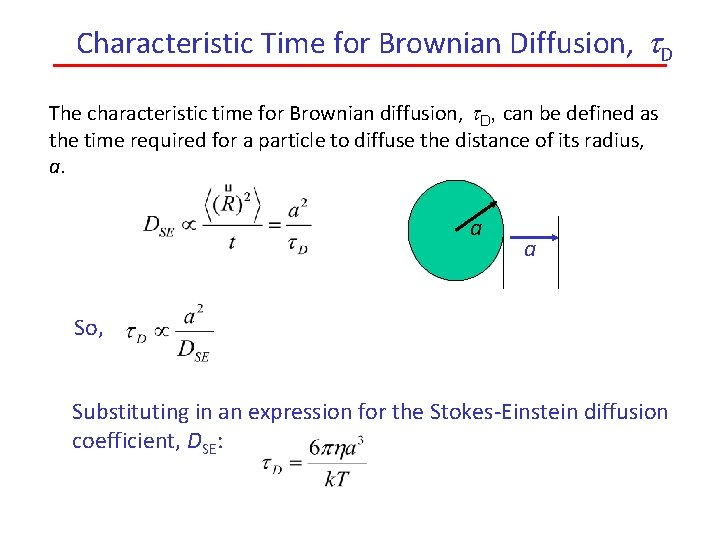Characteristic Time for Brownian Diffusion, t. D The characteristic time for Brownian diffusion, t. D, can be defined as the time required for a particle to diffuse the distance of its radius, a. a a So, Substituting in an expression for the Stokes-Einstein diffusion coefficient, DSE:Competition between Shear Ordering and Brownian Diffusion: Peclet Number, Pe To determine the relative importance of diffusion and shear strain in influencing the structure of colloidal dispersions, we can compare their characteristic times through a Peclet number: (a unitless parameter) Substituting in values for each characteristic time: Thus, when Pe >1, diffusion is slow (t. D is long) relative to the time of shear strain (t. S). Hence, the shear stress can order the particles and lower the h. Shear thinning is observed!A “Universal” Dependence of h on Pe Data for different colloids of differing size and type Shear thinning region Small a; Low Large a; High When Pe <1, t. D is short in comparison to t. S, and the particles are not ordered because Brownian diffusion randomises them.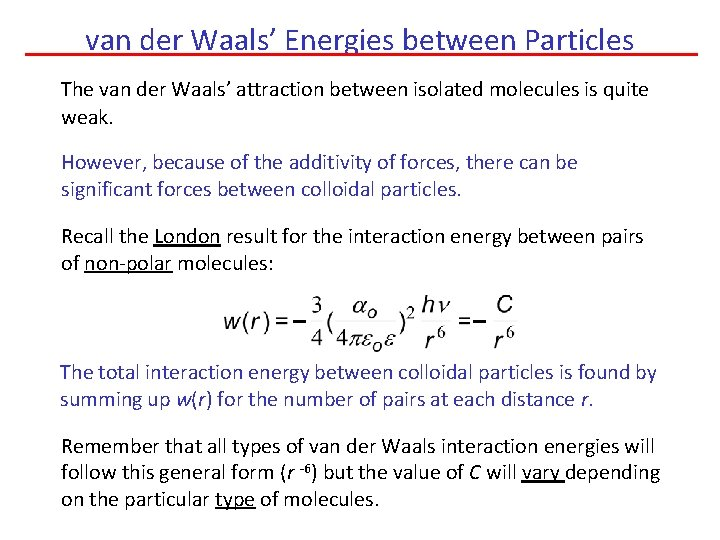van der Waals’ Energies between Particles The van der Waals’ attraction between isolated molecules is quite weak. However, because of the additivity of forces, there can be significant forces between colloidal particles. Recall the London result for the interaction energy between pairs of non-polar molecules: The total interaction energy between colloidal particles is found by summing up w(r) for the number of pairs at each distance r. Remember that all types of van der Waals interaction energies will follow this general form (r -6) but the value of C will vary depending on the particular type of molecules.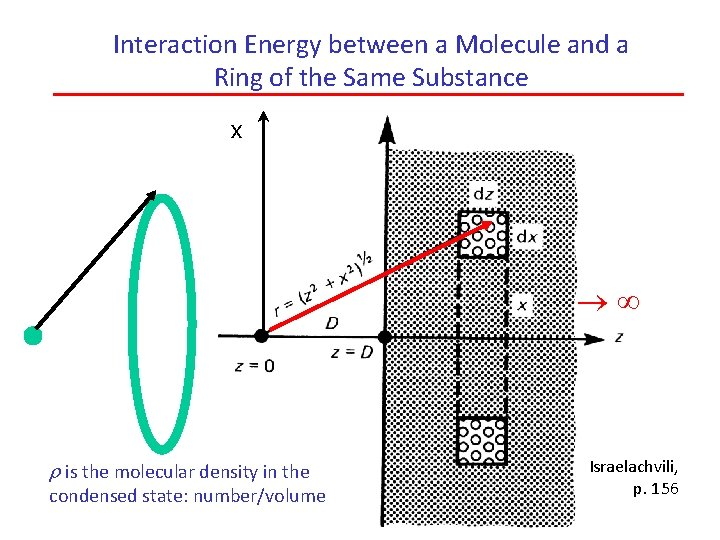Interaction Energy between a Molecule and a Ring of the Same Substance x r is the molecular density in the condensed state: number/volume Israelachvili, p. 156Interaction Energy between a Molecule and a Ring of the Same Substance The cross-sectional area of the ring is dxdz. The volume of the ring is thus V = 2 pxdxdz. If the substance contains r molecules per unit volume in the condensed phase, then the number of molecules in the ring is N = r. V = 2 prxdxdz. The distance, r, from the molecule to the ring is: The total interaction energy between the molecule and N molecules in the ring can be written as:Interaction Energy between a Molecule and a Slab of the Same Substance x Semi- slab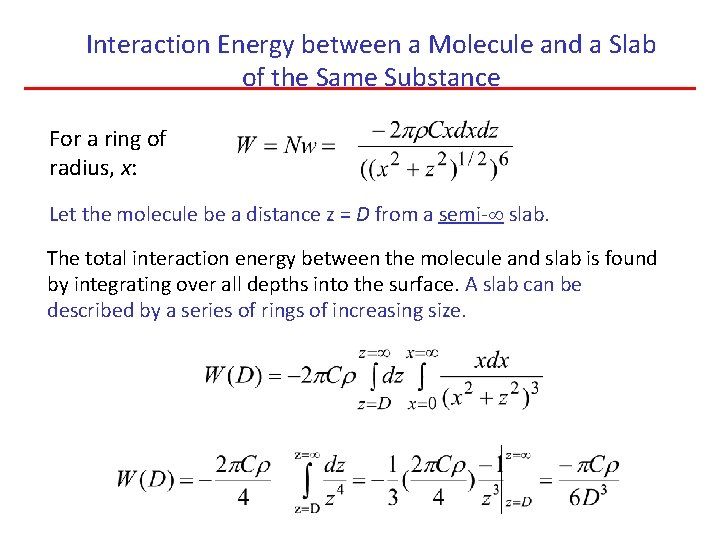Interaction Energy between a Molecule and a Slab of the Same Substance For a ring of radius, x: Let the molecule be a distance z = D from a semi- slab. The total interaction energy between the molecule and slab is found by integrating over all depths into the surface. A slab can be described by a series of rings of increasing size.Attractive Force between a Molecule and a Slab of the Same Substance • D Force is obtained from the derivative of energy with respect to distance:Interaction Energy between a Particle and a Slab of the Same Substance x z =0 z =2 R R x D D+z z dz Slice Thickness = dz 2 R-z z R = particle radius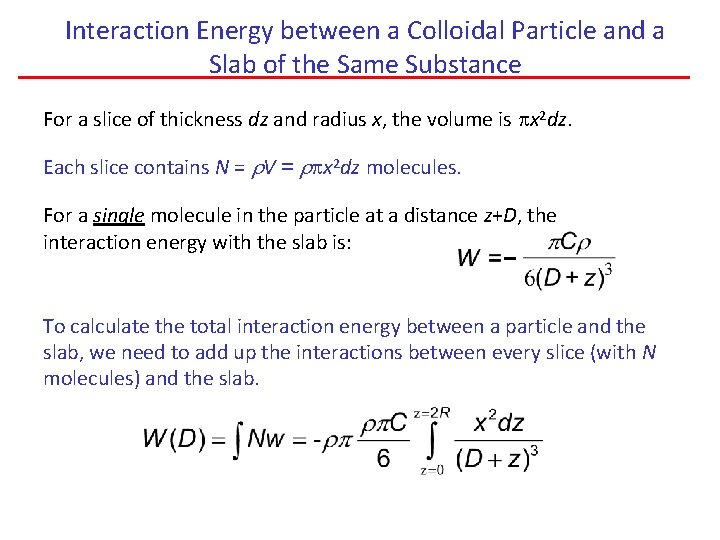Interaction Energy between a Colloidal Particle and a Slab of the Same Substance For a slice of thickness dz and radius x, the volume is px 2 dz. Each slice contains N = r. V = rpx 2 dz molecules. For a single molecule in the particle at a distance z+D, the interaction energy with the slab is: To calculate the total interaction energy between a particle and the slab, we need to add up the interactions between every slice (with N molecules) and the slab.Interaction Energy between a Colloidal Particle and a Slab of the Same Substance 0 For a sphere with a radius of R, the chord theorem tells us that x 2 = (2 R - z)z. Substituting in for x 2: But if D<<R, which is the case for close approach when vd. W forces are active, only small values of z contribute significantly to the integral, and so integrating up to z = will not introduce much error. We can also neglect the z in the brackets in the numerator because z <<R when energies are large. 0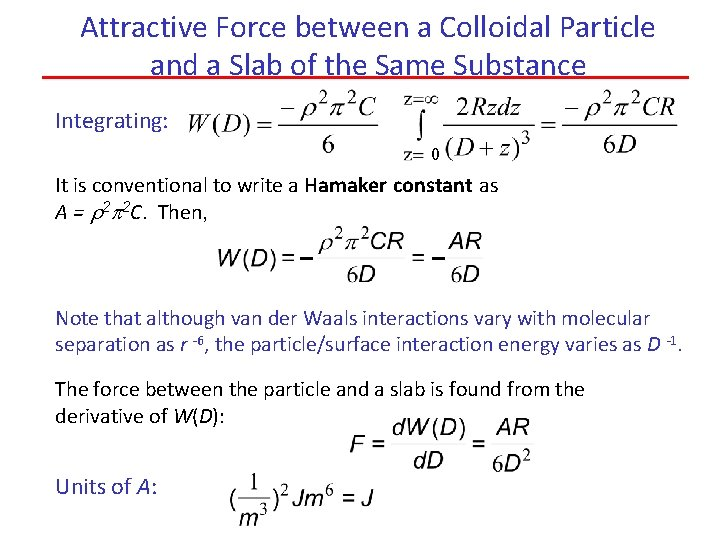Attractive Force between a Colloidal Particle and a Slab of the Same Substance Integrating: 0 It is conventional to write a Hamaker constant as A = r 2 p 2 C. Then, Note that although van der Waals interactions vary with molecular separation as r -6, the particle/surface interaction energy varies as D -1. The force between the particle and a slab is found from the derivative of W(D): Units of A:Hamaker Constants for Identical Substances Acting Across a Vacuum A = p 2 Cr 2 Substance Hydrocarbon C (10 -79 Jm 6) r (1028 m-3) A (10 -19 J) 50 3. 3 0. 5 CCl 4 1500 0. 6 0. 5 H 2 O 140 3. 3 1. 5 “A” tends to be about 10 -19 J for many substances. Why? For non-polar, uncharged molecules, recall the definition of the London constant: C o 2 If v = molecular volume, we know that r 1/v and ao r 3 v So, roughly we see: A Cr 2 o 2 r 2 v 2/v 2 = a constant!Surface-Surface Interaction Energies The attractive energy between two semi- planar slabs is ! Can consider the energy between a unit area (A) of surface and a semi - slab. dz D z z=0 Unit area In a slice of thickness dz, there are N =r. Adz molecules. In a unit area, A = 1, and N = rdz. We recall that for a single molecule: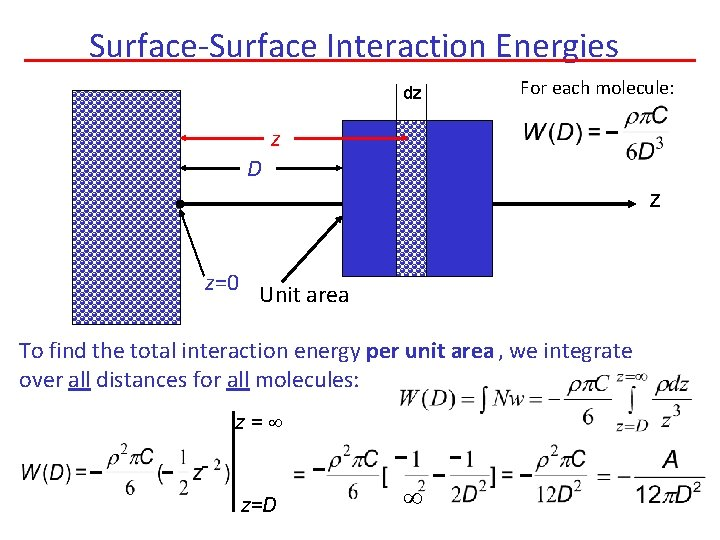Surface-Surface Interaction Energies dz For each molecule: z D z z=0 Unit area To find the total interaction energy per unit area , we integrate over all distances for all molecules: z= z=D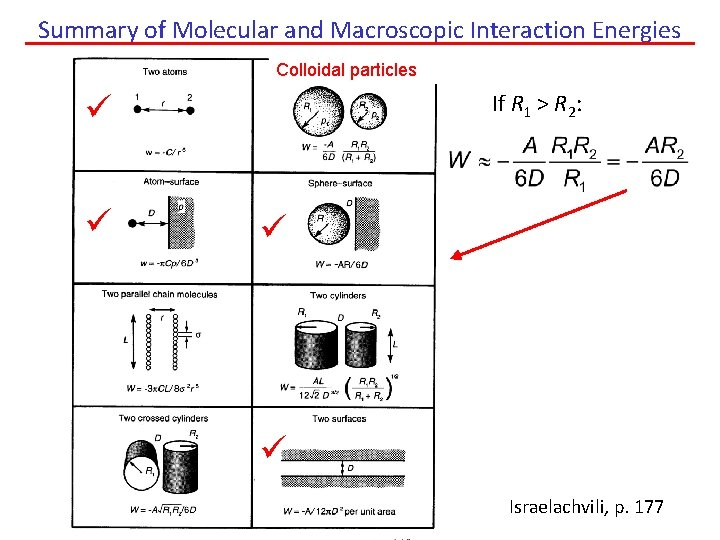Summary of Molecular and Macroscopic Interaction Energies Colloidal particles If R 1 > R 2: Israelachvili, p. 177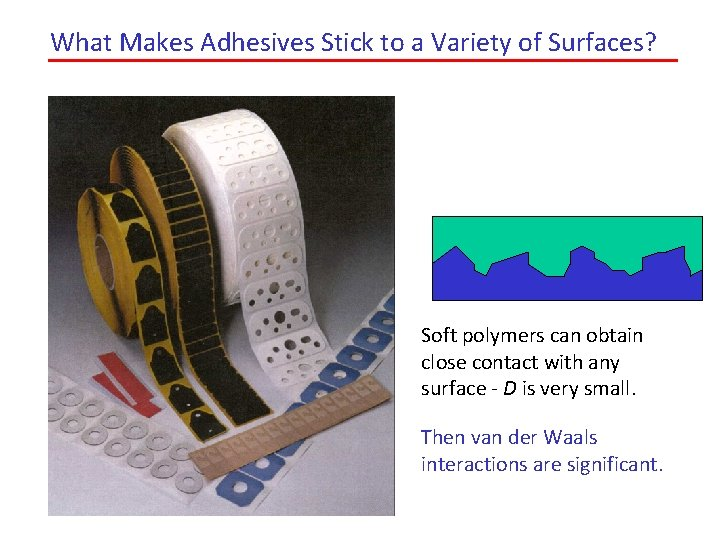What Makes Adhesives Stick to a Variety of Surfaces? Soft polymers can obtain close contact with any surface - D is very small. Then van der Waals interactions are significant.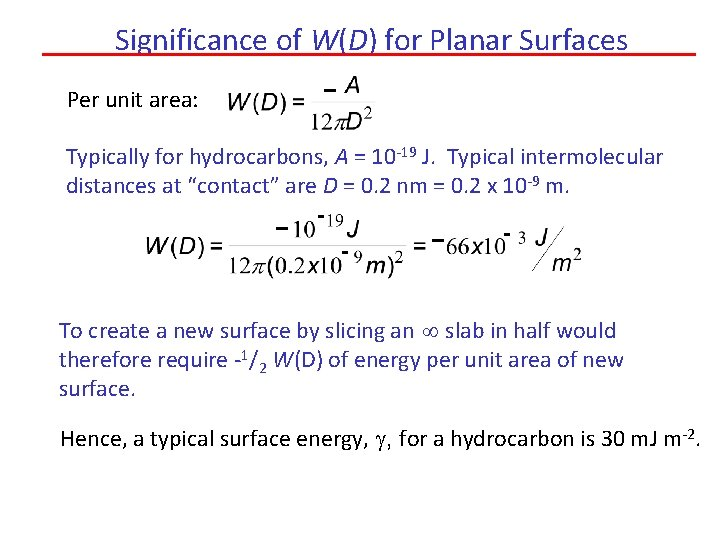Significance of W(D) for Planar Surfaces Per unit area: Typically for hydrocarbons, A = 10 -19 J. Typical intermolecular distances at “contact” are D = 0. 2 nm = 0. 2 x 10 -9 m. To create a new surface by slicing an slab in half would therefore require -1/2 W(D) of energy per unit area of new surface. Hence, a typical surface energy, g, for a hydrocarbon is 30 m. J m-2.Adhesion Force for Planar Surfaces As we’ve seen before, the force between two objects is F = d. W/d. D, so for two planar surfaces we find: As W is per unit area, the force is likewise per unit area. Thus, it has the dimensions of pressure, (P = F/A) Using typical values for A and assuming “molecular contact”: This pressure corresponds to nearly 7000 atmospheres! But it requires very close contact.Gecko’s stick to nearly any surface – even under water – because of van der Waals attractive forces A Gecko Pads on feet Spatulae Setae Images from http: //news. bbc. co. uk/1/hi/sci/tech/781611. stmSynthetic “Gecko” Tape When polymer fibers make close contact to surfaces, they adhere strongly. But van der Waals’ forces also cause attraction between the fibers!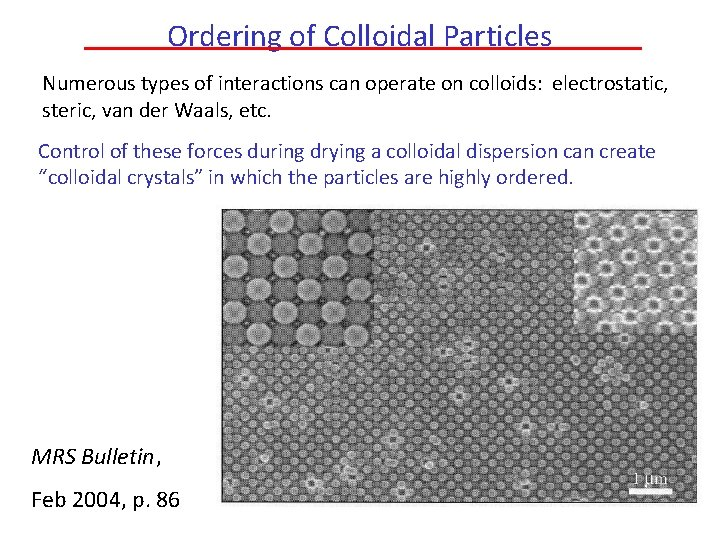Ordering of Colloidal Particles Numerous types of interactions can operate on colloids: electrostatic, steric, van der Waals, etc. Control of these forces during drying a colloidal dispersion can create “colloidal crystals” in which the particles are highly ordered. MRS Bulletin, Feb 2004, p. 86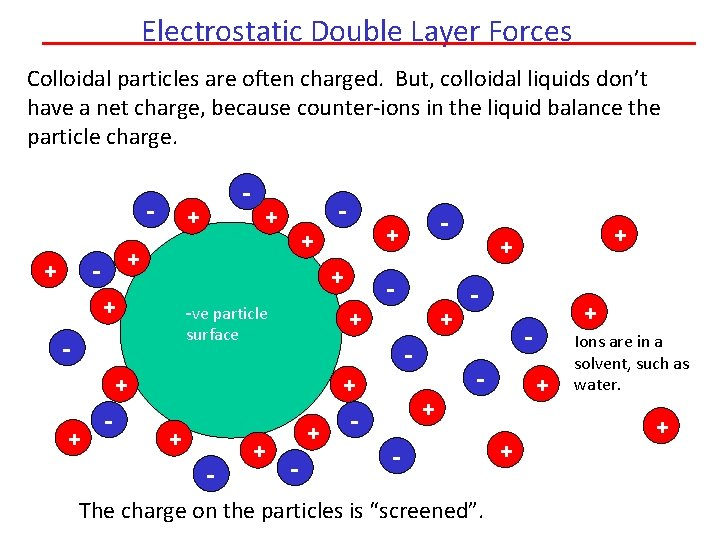Electrostatic Double Layer Forces Colloidal particles are often charged. But, colloidal liquids don’t have a net charge, because counter-ions in the liquid balance the particle charge. - + + + -ve particle surface - + + + - - + + The charge on the particles is “screened”. + Ions are in a solvent, such as water. +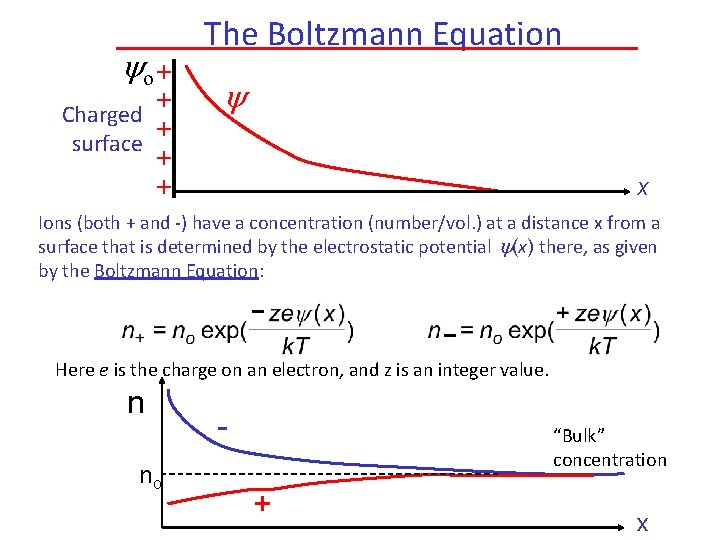o + + Charged + surface + + The Boltzmann Equation x Ions (both + and -) have a concentration (number/vol. ) at a distance x from a surface that is determined by the electrostatic potential (x) there, as given by the Boltzmann Equation: Here e is the charge on an electron, and z is an integer value. n no - “Bulk” concentration + xThe Poisson Equation In turn, the spatial distribution of the electrostatic potential is described by the Poisson equation: The net charge density, r, (in the simple case in which there are only ions to counter-balance the surface charge) is But n+ and n- can be given by the Boltzmann equation, and then the Poisson-Boltzmann equation is obtained:Solutions of the Poisson-Boltzmann Equation We recall that So, The P-B Equation then becomes: But when x is small, sinh(x) x, and so for small : In this limit, a solution of the P-B equation is where -1 is called the Debye screening length.The Debye Screening Length, -1 - + - - + + + - + + k-1 It can be shown that depends on the ionic (salt) concentration, no, and on the valency, z, as well as e for the liquid (e = 85 for water). For one mole/L of salt in water, -1 = 0. 3 nm. As the salt concentration increases, the distance over which the particle charge acts decreases. x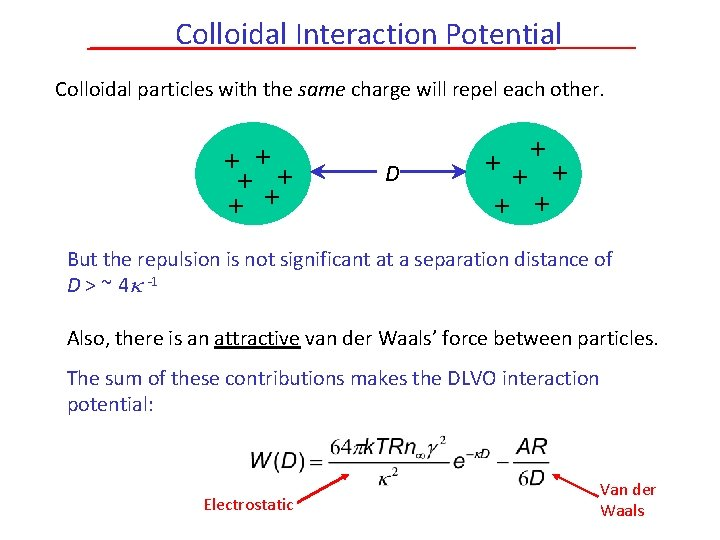Colloidal Interaction Potential Colloidal particles with the same charge will repel each other. + + + D + + + But the repulsion is not significant at a separation distance of D > ~ 4 -1 Also, there is an attractive van der Waals’ force between particles. The sum of these contributions makes the DLVO interaction potential: Electrostatic Van der Waals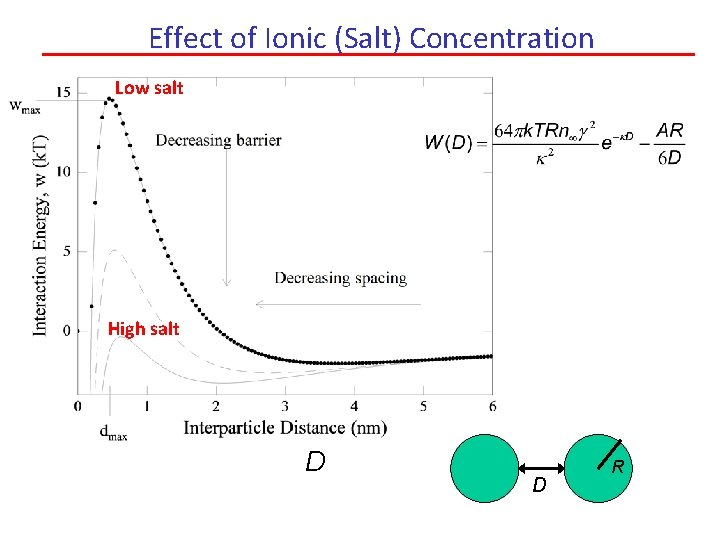Effect of Ionic (Salt) Concentration Low salt High salt D D RPacking of Colloidal Particles When mono-sized, spherical particles are packed into an FCC arrangement, they fill a volume fraction f of 0. 74 of free space. e 4 r r f= Sphere V Occupied V = e 3 Can you prove to yourself that this is true? When randomly-packed, f is typically ~0. 6 for spherical particles. (Interestingly, oblate spheroidal particles (e. g. peanut M&Ms) fill a greater fraction of space when randomly packed!) The Debye screening length can contribute to the effective particle radius and prevent dense particle packing of colloidal particle dispersed in a liquid (e. g. water).Effect of Salt on Packing of Charged Particles 0. 7 Ordered: FCC packing f Disordered Long screening length salt concentration Short screening length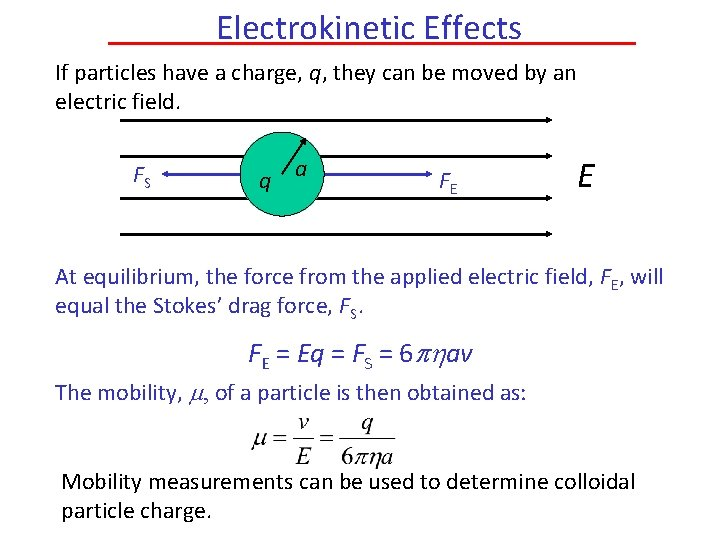Electrokinetic Effects If particles have a charge, q, they can be moved by an electric field. FS q a FE E At equilibrium, the force from the applied electric field, FE, will equal the Stokes’ drag force, FS. FE = Eq = FS = 6 phav The mobility, m, of a particle is then obtained as: Mobility measurements can be used to determine colloidal particle charge.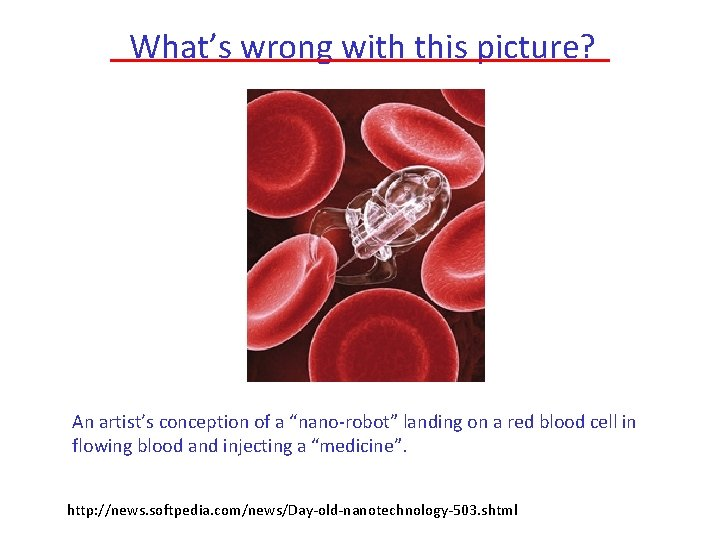What’s wrong with this picture? An artist’s conception of a “nano-robot” landing on a red blood cell in flowing blood and injecting a “medicine”. http: //news. softpedia. com/news/Day-old-nanotechnology-503. shtmlNeed to consider: • Brownian motion – in both the nano-robot and the cells • Drag force – acts on nano-robot and makes it difficult to propel • Attractive surface forces – the nano-robot will be attracted from all sides to cells and other objects. • How to control the robot orientation?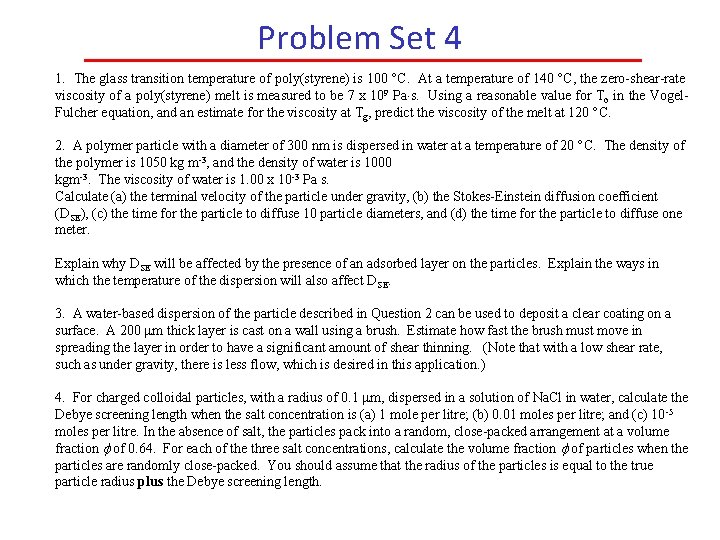Problem Set 4 1. The glass transition temperature of poly(styrene) is 100 C. At a temperature of 140 C, the zero-shear-rate viscosity of a poly(styrene) melt is measured to be 7 x 109 Pa s. Using a reasonable value for To in the Vogel. Fulcher equation, and an estimate for the viscosity at Tg, predict the viscosity of the melt at 120 C. 2. A polymer particle with a diameter of 300 nm is dispersed in water at a temperature of 20 C. The density of the polymer is 1050 kg m-3, and the density of water is 1000 kgm-3. The viscosity of water is 1. 00 x 10 -3 Pa s. Calculate (a) the terminal velocity of the particle under gravity, (b) the Stokes-Einstein diffusion coefficient (DSE), (c) the time for the particle to diffuse 10 particle diameters, and (d) the time for the particle to diffuse one meter. Explain why DSE will be affected by the presence of an adsorbed layer on the particles. Explain the ways in which the temperature of the dispersion will also affect DSE. 3. A water-based dispersion of the particle described in Question 2 can be used to deposit a clear coating on a surface. A 200 mm thick layer is cast on a wall using a brush. Estimate how fast the brush must move in spreading the layer in order to have a significant amount of shear thinning. (Note that with a low shear rate, such as under gravity, there is less flow, which is desired in this application. ) 4. For charged colloidal particles, with a radius of 0. 1 mm, dispersed in a solution of Na. Cl in water, calculate the Debye screening length when the salt concentration is (a) 1 mole per litre; (b) 0. 01 moles per litre; and (c) 10 -5 moles per litre. In the absence of salt, the particles pack into a random, close-packed arrangement at a volume fraction f of 0. 64. For each of the three salt concentrations, calculate the volume fraction f of particles when the particles are randomly close-packed. You should assume that the radius of the particles is equal to the true particle radius plus the Debye screening length.Problem Set 4 1. The glass transition temperature of poly(styrene) is 100 C. At a temperature of 140 C, the zero-shear-rate viscosity of a poly(styrene) melt is measured to be 7 x 109 Pa s. Using a reasonable value for To in the Vogel-Fulcher equation, and an estimate for the viscosity at Tg, predict the viscosity of the melt at 120 C. 2. A polymer particle with a diameter of 300 nm is dispersed in water at a temperature of 20 C. The density of the polymer is 1050 kg m-3, and the density of water is 1000 kgm-3. The viscosity of water is 1. 00 x 10 -3 Pa s. Calculate (a) the terminal velocity of the particle under gravity, (b) the Stokes-Einstein diffusion coefficient (DSE), (c) the time for the particle to diffuse 10 particle diameters, and (d) the time for the particle to diffuse one meter. Explain why DSE will be affected by the presence of an adsorbed layer on the particles. Explain the ways in which the temperature of the dispersion will also affect DSE. 3. A water-based dispersion of the particle described in Question 2 can be used to deposit a clear coating on a surface. A 200 mm thick layer is cast on a wall using a brush. Estimate how fast the brush must move in spreading the layer in order to have a significant amount of shear thinning. (Note that with a low shear rate, such as under gravity, there is less flow, which is desired in this application. )